Number Bases - Binary

How Data is Stored in Computers

As we have seen before, computers rely on a sequence of switches which can be in one of two states - ON or OFF.

To make things simpler, computer scientists often think of these switches in terms of 1s and 0s:

1. 1 is ON
2. 0 is OFF

This allows computer scientists to represent a sequence of switches using a number consisting of 0s and 1s (known as binary). For example, the sequence 1001 would represent switches being__ ON-OFF-OFF-ON__.

Each one of these binary digits is known as a bit, and is represented by a b.

e.g. 010 is a 3-bit number

We also have slightly longer binary numbers:

1. 4 bits = 1 Nibble (e.g. 1001)
2. 8 bits = 1 Byte (e.g. 1010 1000)

If we have 1B (B = Byte) of data, this means that we have 8b (b = bits) of data - a binary number with 8 digits. However, since 1B of data is only enough to store 1 character on a computer, we need to have much larger sizes to describe data that is actually stored.

1. 1000B = 1 kilobyte = 1kB
2. 1000kB = 1 megabyte = 1MB
3. 1000MB = 1 gigabyte = 1GB
4. 1000GB = 1 terabyte = 1TB

To get an idea of these sizes:

1. A page of text is about 2 kB
2. 1MB is about 1 minute of MP3 audio
3. A DVD is around 4-8GB
4. 1TB of data is enough to fill 1,428 CDs

Number Bases - Binary

There are 10 types of people in this world; those who understand binary and those who do not!

When you first start to learn to count, your teacher tells you to use your fingers. This is great until we reached 10… once numbers started getting bigger than 9, we have to start all over again at 0 and simply remember how many times we have got to 10.

Our counting system we use still uses this method of counting. For example, the number 23 means that we have counted up to 10 twice, and have 3 units left over. Again though we have a problem when we reach 99 and we have to add a digit on the front, and have a new column called ‘hundreds’. In fact, we represent this using the units, tens, hundreds, thousands headings.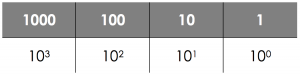Each time we move one to the left, we multiply that place value by 10.

Any base number system uses this method, but the numbers that we use to count changes each time. For example, in decimal (normal counting system) we have 10 digits (0-9) whilst in binary (counting system used by computers) we have 2 digits (0 and 1) and in hexadecimal (another system you will become familiar with!) we have 16 digits (0-9A-F).

Binary to Decimal (or Denary)

When converting from binary to decimal, we first write our headings that we have above. Then working from the right, we put the bits we have into each box.

Example - Convert 0101 0110 into decimal:

1. Write the place headings above.
2. Put the numbers under the place headings.
4. This is your decimal value.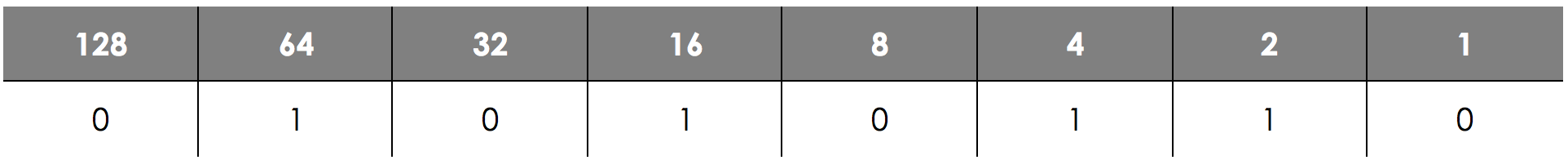1. 64 + 16 + 4 + 2 = 86
2. Therefore 0101 0110 in decimal is 86.

Converting Binary Numbers

Since we are able to communicate with computers using this system where 0 and 1 represent OFF and ON, this allows us to create numbers to represent different things on the computer system - for example, text, an image or even sound. However, working with binary numbers can be long and difficult, so we need to be able to convert between binary and our normal decimal (or denary) counting system.

To do this, we need to remember that binary is Base 2. This means that our place headings in binary multiply by 2 each time we move 1 space to the left.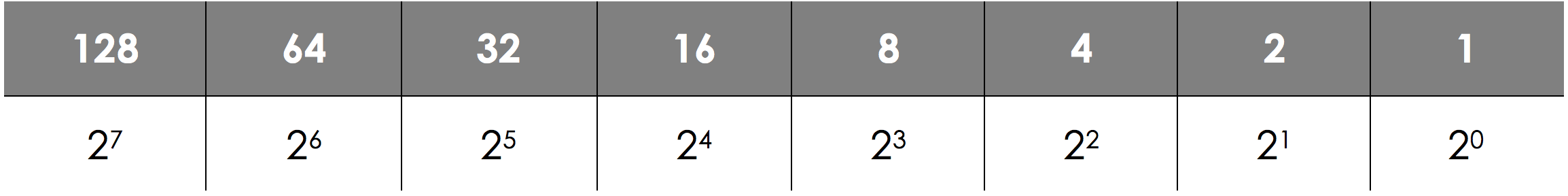Decimal (or Denary) to Binary

There are two different methods for doing this - you should pick the one you are most comfortable with and stick with it!

Method 1 - Subtraction

Draw the grid out with the column headers as before, and starting from the largest number on the left, work out which number is the biggest number that can be taken away from it. In that column, write a 1. Then, take that number away from the original, and keep repeating until you have nothing left.

Example - Convert 86 into binary

Write the place headings above. Find the largest number that goes into 86 and put a 1 in that column - in this case it’s 64.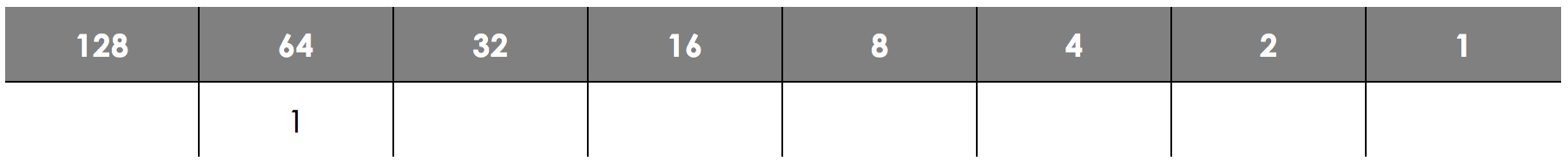Now take 64 away from the original 86:

86 - 64 = 22

Now, working right from 64, work out which the next biggest heading is - in this case it is 16.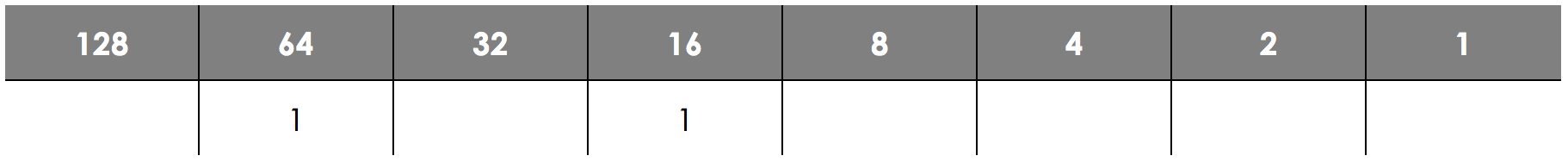Now take 16 away from 22:

22 - 16 = 6

Again, working right from 16, the next biggest heading that goes into 6 is 4.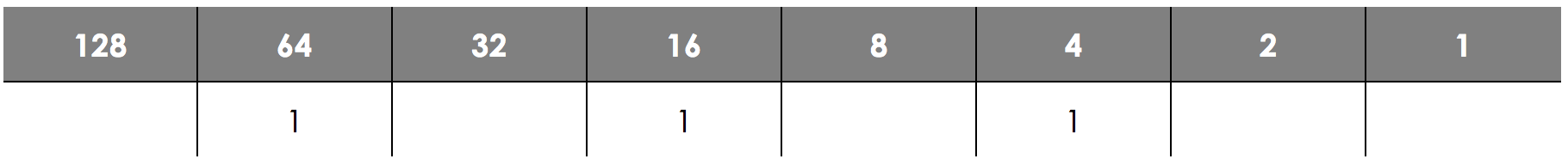Now take 4 away from 6:

6 - 4 = 2

Again, the next biggest heading that goes into 2 is 2.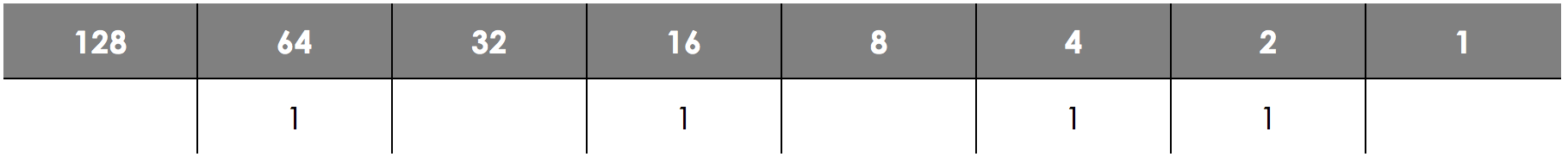Finally, we take 2 away from 2 and get to 0:

2 - 2 = 0

Since we have now run out of numbers, we simply fill in the rest of the table with 0s.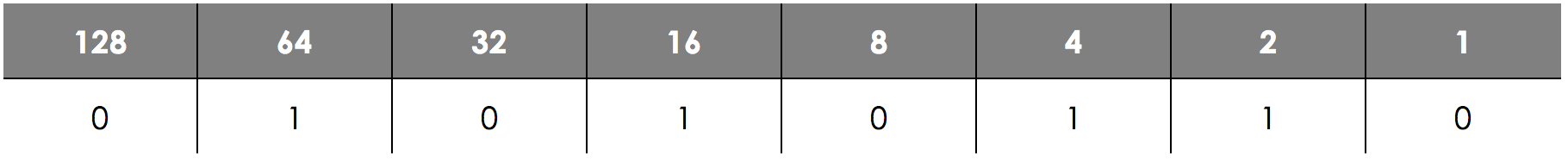This means that 86 in binary is 0101 0110.

Method 2 - Division

There is an alternative method using division by 2. Keep dividing the whole part of the division by 2 and the remainders will then be the binary number.

Example - Convert 86 into binary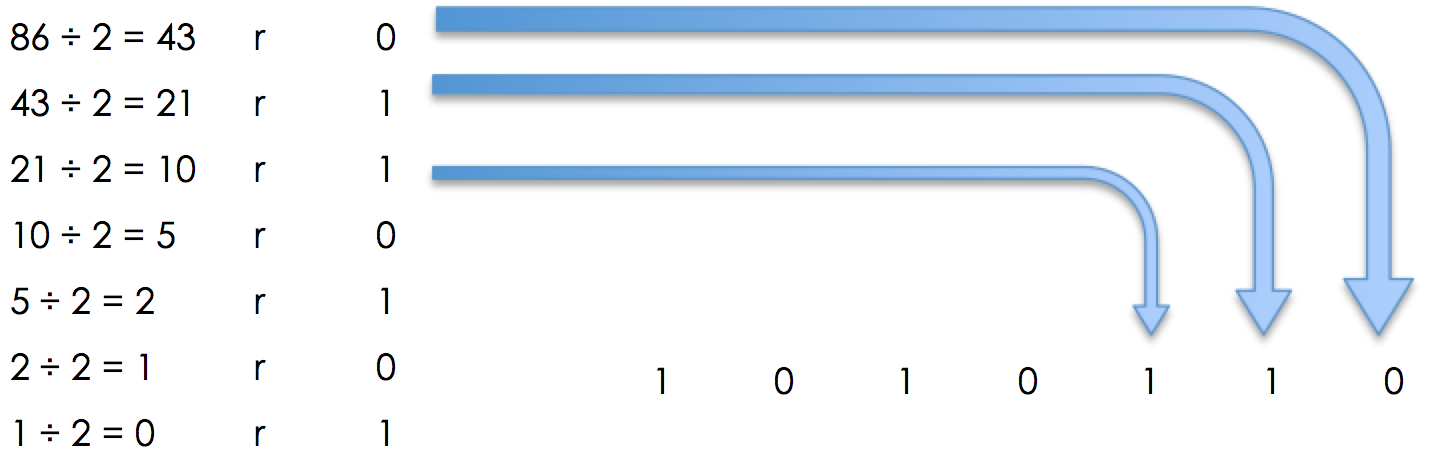Each time you divide by 2, the remainder is placed into the final number. So here, we have that 86 in binary is 101 0110 - notice that in this method, we may need to add the zeros to the front of the number to get a full byte.

Convert these units of information (don't include the units):2500 bytes into kilobytes:
2.5
Convert these units of information (don't include the units):0.97 terabytes into gigabytes
970
Convert these units of information (don't include the units):7,300,000 megabytes into terabytes?
7.3
Convert the following binary numbers into decimal:0000 1001
9
Convert the following binary numbers into decimal:1110 1101
237
Convert the following binary numbers into decimal:0111 1010
122
Convert the following decimal numbers into binary (don't add spaces):37
00100101
Convert the following decimal numbers into binary (don't add spaces):154
10011010
Convert the following decimal numbers into binary (don't add spaces):229
11100101